# 盛世娇宠：听说皇上惧内

## 正文第十章 别忘了自己是谁

[更新时间] 2018-09-26 15:39:31 [字数] 2022

==&^+本=书首!发纵=横=中文网^=@^*

“二哥？”==&^+本=书首!发纵=横=中文网^=@^*

“婉茹，这是五弟沐辰，想必你应该不陌生吧？”==&^+本=书首!发纵=横=中文网^=@^*

“五弟，不该是你的，你强求也没用，这几日朕会为你赐婚的，你年纪也不小了，该成婚了。”==&^+本=书首!发纵=横=中文网^=@^*

“二哥，我不会成婚的，除了她之外，别人我都不要，所以，你要是想让我成婚的话，就将她赐给我吧。”==&^+本=书首!发纵=横=中文网^=@^*

“她，你就不用想了，她已经是我的女人了，以后也只能是我的女人，不要妄想那些本就不属于你的东西，注定是你的，跑不掉，注定不是你的，你强求也没用。”==&^+本=书首!发纵=横=中文网^=@^*

“时候不早了，朕还有要事处理，爱妃，咱们回宫吧。”==&^+本=书首!发纵=横=中文网^=@^*

“快点回宫。”==&^+本=书首!发纵=横=中文网^=@^*

“唔……你发什么疯啊？”==&^+本=书首!发纵=横=中文网^=@^*

“爱妃，你不是说要给朕侍寝吗？那就现在吧。”==&^+本=书首!发纵=横=中文网^=@^*

“我……现在还是白天，你不能这样，你……”==&^+本=书首!发纵=横=中文网^=@^*

“啊……不要啊”，刚说完，林婉茹的身体就已经被贯穿了，而且身上的男人此刻眼神中除了愤怒，就只剩下占有了。==&^+本=书首!发纵=横=中文网^=@^*

“不要，你放开我，你……”==&^+本=书首!发纵=横=中文网^=@^*

“怎么？一看见你的心上人，就不想让朕宠爱你了？”==&^+本=书首!发纵=横=中文网^=@^*

“你别忘了，如今你已经是朕的女人了，也只有朕才配享用你的滋味。”==&^+本=书首!发纵=横=中文网^=@^*

“今日朕给你留下这个印记，只是提醒你，别忘了自己是谁的女人，若是日后再让朕看到你跟任何男人之间有什么眉目传情的举动，就不是这么简单了。”==&^+本=书首!发纵=横=中文网^=@^*

“紫鸢，你去给我弄碗粥过来吧，然后再准备一桶水，我要沐浴。”==&^+本=书首!发纵=横=中文网^=@^*

==&^+本=书首!发纵=横=中文网^=@^*

(快捷键：←)上一章 回目录(快捷键：回车) 下一章(快捷键：→)

### 发表评论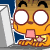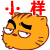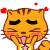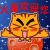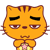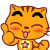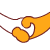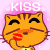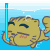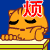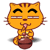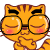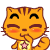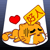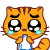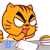12